### Vectors and their Operations: Cross product

### The cross product and its properties

The cross product of two vectors is an operation that takes two vectors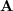and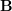and returns a vector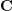. This operation is denoted by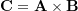and is read “C equals A cross B”. The magnitude of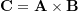is defined as,

(2.22)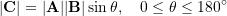in which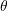is the angle between the two vectors.

The definition of the magnitude of the cross product implies that,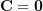if two non-zero vectors are parallel.

By definition,is perpendicular to bothand, which are not parallel, and its sense of direction is specified by the right-hand rule. In other words,is perpendicular to the plane containing the non-parallel vectorsandsuch that(or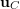) is in the direction of the thumb following the right-hand rule (Fig. 2.26a). The right-hand rule can also be equivalently demonstrated by the right-hand three-finger rule as demonstrated in Fig. 2.26b.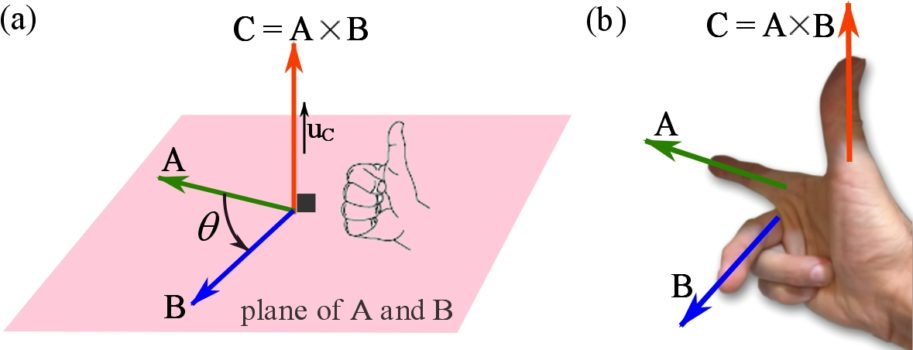Fig. 2.26  (a) The right-hand rule and (b) the right-hand three-finger rule for determining the direction of a cross product.

Ifis the unit vector of, the resultant of a cross product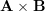can be written as,

(2.23)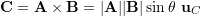The following interactive tool illustrates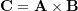. Use the sliders to change the angles ofandwith the Cartesian axes, and observe the resultant of their cross product.

##### Properties of Cross product
1. Anti-commutativity: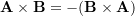2. Associativity: forbeing a scalar,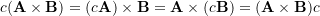3. Distributivity: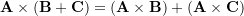### Cross product in CVN

In a Cartesian frame the following relationships are readily obtained for the mutually perpendicular unit vectors,and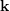.

(2.24)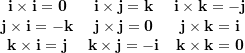Based on the above results and the properties of the cross product, the cross product of two vectors in their CVN is,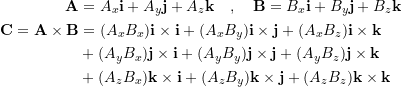(2.25)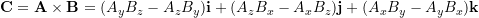This result need not to be memorized and can be obtained by calculating the following determinant symbolically.

(2.26)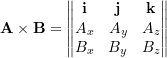As a reminder, the determinant of a two-by-two matrix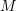is a scalar defined as,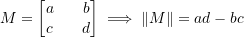Consequently, the determinant of a three-by-three matrixis a scalar defined as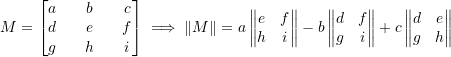The following tool calculates the cross product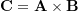. The vectoris drawn in green.